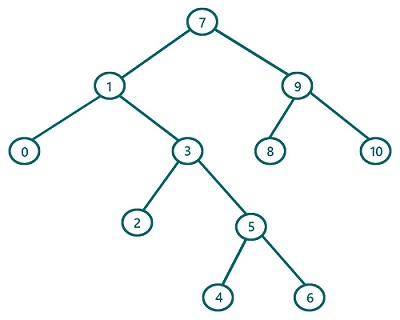# Preorder Traversal in Binary Tree (with recursion) in C, C++

In this article, we are going to find what preorder traversal of a Binary Tree is and how to implement preorder traversal using recursion? We have provided the implementation both in C & C++.
Submitted by Radib Kar, on July 24, 2020

If we classify tree traversals, preorder traversal is one of the traversal techniques which is based on depth-first search traversal.  The basic concept for preorder traversal lies in its name itself. Pre means before (first/initially) and that's why root is being traversed first and then the subtrees. So, the rule is:

1. First, traverse the root
2. Then traverse the left subtree
3. Finally, traverse the right subtree

Of course, while traversing the subtrees we will follow the same order

So let's traverse the below tree using preorder traversal.For the above tree, the root is: 7

So first print root -> 7

Traversal till now: 7

Then Traverse the left subtree (Subtree rooted by 1)

Now to traverse the subtree rooted by 1, it again follows the same set of rules

So First print 1

Traversal till now: 7 1

Then traverse the left subtree of this (rooted by 0)

Since it's a leaf node we can print it as there is no more left subtree. Also, 0 has no subtree, so this subtree rooted by 0 is traversed totally.

Traversal till now: 7 1 0

Since the left subtree of the subtree rooted 1 is traversed, so now traverse the right subtree.

After processing right subtree traversal of the tree rooted by 1 we will have traversal: 7 1 0 3 2 5 4 6

Now we have finished the left subtree traversal of the actual tree (rooted by 7)

Rest is about traversing the right subtree of the root 7. So ultimately after the whole traversal, we will have: 7 1 0 3 2 5 4 6 9 8 10

The pseudocode would be:

```void preorder(TreeNode root){
if(root is NULL)
return
//traverse current node first
print(root)
//recursively traverse the left subtree secondly
preorder (left subtree of the root)
// recursively traverse the right subtree finally
preorder (right subtree of the root)
}
```

C Implementation:

Tree structure:

```struct tree{
int val;
struct tree* left;
struct tree* right;
};

typedef struct tree TreeNode;
```
```#include <stdio.h>
#include <stdlib.h>

struct tree {
int val;
struct tree* left;
struct tree* right;
};

typedef struct tree TreeNode;

TreeNode* newTree(int data)
{
// Allocate memory for new node
TreeNode* root = (TreeNode*)malloc(sizeof(TreeNode));

// Assign data to this node val
root->val = data;

// Initialize left and right children as NULL
root->left = NULL;
root->right = NULL;
return (root);
}

void preorder(TreeNode* root)
{
//base case
if (root == NULL)
return;

//traverse current node first
printf("%d ", root->val);

//traverse left sub tree then
preorder(root->left);

//traverse right sub tree finally
preorder(root->right);
}

int main()
{
//building the tree
TreeNode* t = newTree(7);
t->left = newTree(1);
t->left->left = newTree(0);
t->left->right = newTree(3);
t->left->right->left = newTree(2);
t->left->right->right = newTree(5);
t->left->right->right->left = newTree(4);
t->left->right->right->right = newTree(6);
t->right = newTree(9);
t->right->left = newTree(8);
t->right->right = newTree(10);

printf("Preorder traversal of the above tree is:\n");
preorder(t);

return 0;
}
```

Output:

```Preorder traversal of the above tree is:
7 1 0 3 2 5 4 6 9 8 10
```

C++ implementation:

```#include <bits/stdc++.h>
using namespace std;

class TreeNode { // tree node is defined
public:
int val;
TreeNode* left;
TreeNode* right;
TreeNode(int data)
{
val = data;
left = NULL;
right = NULL;
}
};

void preorder(TreeNode* root)
{
//base case
if (root == NULL)
return;

//traverse current node firstly
printf("%d ", root->val);

//traverse left sub tree then
preorder(root->left);

//traverse right sub tree lastly
preorder(root->right);
}

int main()
{
//building the tree
TreeNode* t = new TreeNode(7);
t->left = new TreeNode(1);
t->left->left = new TreeNode(0);
t->left->right = new TreeNode(3);
t->left->right->left = new TreeNode(2);
t->left->right->right = new TreeNode(5);
t->left->right->right->left = new TreeNode(4);
t->left->right->right->right = new TreeNode(6);
t->right = new TreeNode(9);
t->right->left = new TreeNode(8);
t->right->right = new TreeNode(10);

printf("Preorder traversal of the above tree is:\n");
preorder(t);

return 0;
}
```

Output:

```Preorder traversal of the above tree is:
7 1 0 3 2 5 4 6 9 8 10
```

What's New (MCQs)

Top Interview Coding Problems/Challenges!

Languages: » C » C++ » C++ STL » Java » Data Structure » C#.Net » Android » Kotlin » SQL
Web Technologies: » PHP » Python » JavaScript » CSS » Ajax » Node.js » Web programming/HTML
Solved programs: » C » C++ » DS » Java » C#
Aptitude que. & ans.: » C » C++ » Java » DBMS
Interview que. & ans.: » C » Embedded C » Java » SEO » HR
CS Subjects: » CS Basics » O.S. » Networks » DBMS » Embedded Systems » Cloud Computing
» Machine learning » CS Organizations » Linux » DOS
More: » Articles » Puzzles » News/Updates

© https://www.includehelp.com some rights reserved.